### Home > GC > Chapter 12 > Lesson 12.1.4 > Problem12-36

12-36.
1. Find the surface area of the solids below. Assume that the solid in part (a) is a prism with a regular octagonal base and the pyramid in part (b) is a square-based pyramid. Show all work. Homework Help ✎

1.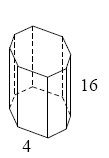2.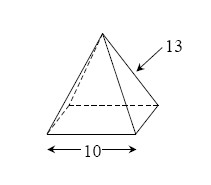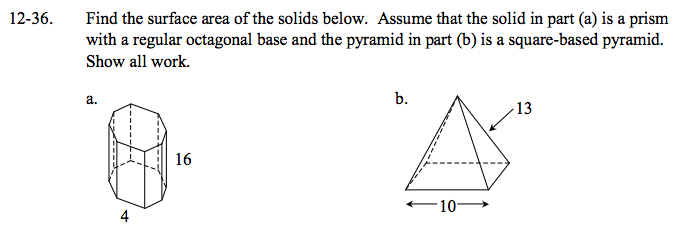Find the area of the top, and the lateral area. Find their sum.

Find the measure of the central angle. Use this to find the area of the triangle. Then use the area to find the total area.

$\text{Center Angle Measure }\frac{ 360^{\circ} }{8 }=45^{\circ}$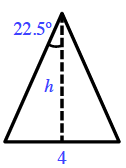$\text{tan}(22.5^{\circ})= \frac{ 2 }{h }$

Area of triangle: A = (0.5)(4)(h) = ?

Use the same method you used to solve part (a).

To find the slant height, draw a diagram.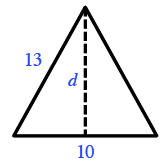Now use the Pythagorean Theorem to find the height.
Use that to find the area of one triangle, and then to
find the total surface area.